Test: Capacitors & Inductors- 2

# Test: Capacitors & Inductors- 2 - Electrical Engineering (EE)

Test Description

## 20 Questions MCQ Test GATE Electrical Engineering (EE) 2024 Mock Test Series - Test: Capacitors & Inductors- 2

Test: Capacitors & Inductors- 2 for Electrical Engineering (EE) 2023 is part of GATE Electrical Engineering (EE) 2024 Mock Test Series preparation. The Test: Capacitors & Inductors- 2 questions and answers have been prepared according to the Electrical Engineering (EE) exam syllabus.The Test: Capacitors & Inductors- 2 MCQs are made for Electrical Engineering (EE) 2023 Exam. Find important definitions, questions, notes, meanings, examples, exercises, MCQs and online tests for Test: Capacitors & Inductors- 2 below.
Solutions of Test: Capacitors & Inductors- 2 questions in English are available as part of our GATE Electrical Engineering (EE) 2024 Mock Test Series for Electrical Engineering (EE) & Test: Capacitors & Inductors- 2 solutions in Hindi for GATE Electrical Engineering (EE) 2024 Mock Test Series course. Download more important topics, notes, lectures and mock test series for Electrical Engineering (EE) Exam by signing up for free. Attempt Test: Capacitors & Inductors- 2 | 20 questions in 60 minutes | Mock test for Electrical Engineering (EE) preparation | Free important questions MCQ to study GATE Electrical Engineering (EE) 2024 Mock Test Series for Electrical Engineering (EE) Exam | Download free PDF with solutions
 1 Crore+ students have signed up on EduRev. Have you?
Test: Capacitors & Inductors- 2 - Question 1

### There inductances, 1 H, 2 H and L H are in parallel. The maximum value of equivalent inductance will be

Detailed Solution for Test: Capacitors & Inductors- 2 - Question 1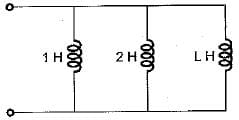Equivalent inductance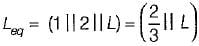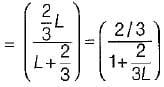Leq will be maximum of L → ∞
Hence,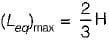Test: Capacitors & Inductors- 2 - Question 2

### Figure given below shows a parallel combination of 10 mH and 20 mH inductances in parallel. A time varying voltage is applied across the inductances at any time instant and the total current supplied by the time varying source is 25 amps. The values of instantaneous currents through each of the inductances are given by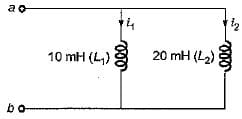Detailed Solution for Test: Capacitors & Inductors- 2 - Question 2

Let the applied voltage be v.
∴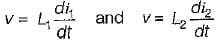or,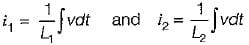∴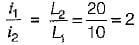or, i1 = 2 i2   ....(i)
Also, i1 + i2 = 25  ...(ii)
Solving equations (i) and (ii), we get
i= 16.67 A and i2 = 8.33A

Test: Capacitors & Inductors- 2 - Question 3

### The equivalent capacitance between the terminals a-b for the capacitive circuit shown below is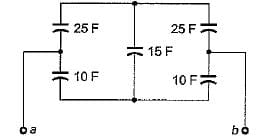Detailed Solution for Test: Capacitors & Inductors- 2 - Question 3

The given circuit can be redrawn as shown below.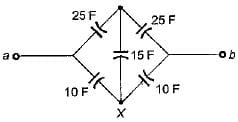The star at point X can be replaced by equivalent delta having delta brances as C1 , C2 and C3 as shown below.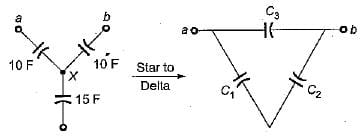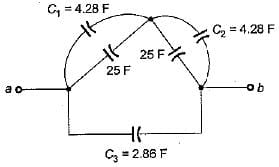Hence,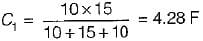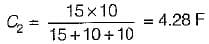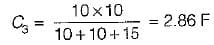Combining the parallel capacitances, the equivalent circuit reduces as shown below.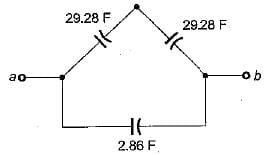Hence, equivalent capacitance between terminals a and b is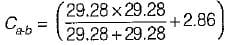= 17.5 F

Test: Capacitors & Inductors- 2 - Question 4

A voltage v(t) = e-t sin t is connected to a capacitance C = 2 F. The energy stored in the capacitor for t = 0 to t = 10 sec is

Detailed Solution for Test: Capacitors & Inductors- 2 - Question 4

Capacitive energy =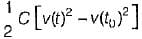Given, v(t) = sin t · e-t
∴ v(10) = (sin 10) · e-10 V
and v(0) = 0 V
∴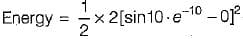= sin210 · e-20 Joules

Test: Capacitors & Inductors- 2 - Question 5

A sawtooth voltage as shown in figure below is applied between the terminals A-B to a capacitor of C = 30 μF. The current i will be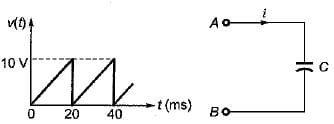Detailed Solution for Test: Capacitors & Inductors- 2 - Question 5

Given waveform is a periodic function having a period of 20 ms.
Here,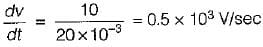∴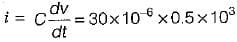= 15 mA

Test: Capacitors & Inductors- 2 - Question 6

Assertion (A): In steady state condition, the energy consumed is infinite for both inductor and capacitor.
Reason (R): The current i in the capacitor and voltage v\n the inductor are zero in steady state.

Detailed Solution for Test: Capacitors & Inductors- 2 - Question 6

Assertion is false because energy consumed is zero in steady state since capacitor acts as open circuit (i = 0) and inductor acts as short-circuit (v = 0) in steady state.

Test: Capacitors & Inductors- 2 - Question 7

Assertion (A): If the voltage across the capacitor being constant, current through it is zero.
Reason (R): On application of dc voltage and with no initial charge, the capacitor first acts as short circuit but as soon as the full charge it retains, the capacitor behaves as open circuit.

Test: Capacitors & Inductors- 2 - Question 8

A 10 μF capacitor is charged full by 100 V dc source. It is then connected across an uncharged capacitor of 50 μF capacity. The energy stored in the two capacitors in the final state will be

Detailed Solution for Test: Capacitors & Inductors- 2 - Question 8

Given, C1 = 10μF
and C2 = 50 μF; V = 100 volt
Initial energy stored in the capacitor of 10 μF is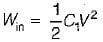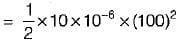= 0.05 Joules
The initial charge,
Qin = CV = 10 x 10-6 x 100
= 0.001 Coulomb
When this 10 μF capacitor is connected across the unchanged capacitor, let the final voltage be V' volt.
Then,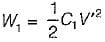and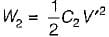Again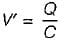where, Q is the final charge and C is the final capacitance.
Now, Q = Qin = 0.001 Columb
and Ceq = C - C1 + C2
= 10 + 50 = 60 μF
Hence, final voltage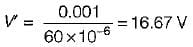Thus,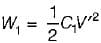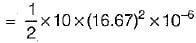= 0.0014 J = 1.4 mJ
and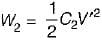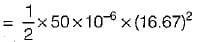= 0.007 J = 7 mJ

Test: Capacitors & Inductors- 2 - Question 9

For the bridge circuit shown below, the charge accumulated in the capacitor under steady state condition is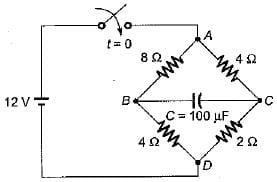Detailed Solution for Test: Capacitors & Inductors- 2 - Question 9

Under steady state condition, the capacitor will acts as open circuit.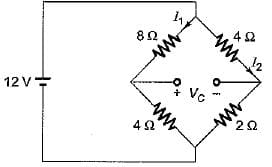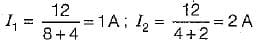Let the voltage across capacitor be VC in steady state.
Then, VC = - 8I1 + 4I2
= - 8 x 1 + 4 x 2
= - 8 + 8 = 0
Hence, charge accumulated in the capacitor is
Q = CV= (100 μF) x 0 = 0

Test: Capacitors & Inductors- 2 - Question 10

A current of i = 300t is suddenly applied to an inductance of 1 mH. The induced voltage will be

Detailed Solution for Test: Capacitors & Inductors- 2 - Question 10

Induced voltage,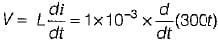= 10-3 x 300 - 0.3 volt

Test: Capacitors & Inductors- 2 - Question 11

A 100-turn coil has an inductance of 6 mH. if the number of turns is increased by 200%, all other quantities remaining the same, the inductance will be

Detailed Solution for Test: Capacitors & Inductors- 2 - Question 11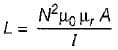∴ L ∝ N2
Since N is increased by 200% i.e. N is doubled therefore, new value of L is
L' = 4 X L
= 4 x 6 = 24 mH

Test: Capacitors & Inductors- 2 - Question 12

A current input,5 δ(t) is flow through a capacitor C. The voltage VC(t), across capacitor is given by

Detailed Solution for Test: Capacitors & Inductors- 2 - Question 12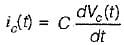or,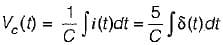Now,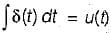So,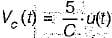Test: Capacitors & Inductors- 2 - Question 13

A voltage waveform v(t) = 12 t2 is applied across1 H inductor for t > 0 with initial current through it being zero. The current through the inductor for t > 0 is given by

Detailed Solution for Test: Capacitors & Inductors- 2 - Question 13

We know that,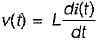or,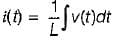or,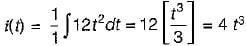Test: Capacitors & Inductors- 2 - Question 14

Which of the following statements associated with capacitor is wrong?

Detailed Solution for Test: Capacitors & Inductors- 2 - Question 14

Statement (b) holds true for an inductor not for a capacitor.

Test: Capacitors & Inductors- 2 - Question 15

Potential difference across a capacitor of capacitance of 20 μF is increased uniformly from 0 to 240 V in 1 second. The charging current will be

Detailed Solution for Test: Capacitors & Inductors- 2 - Question 15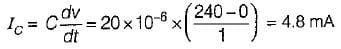Test: Capacitors & Inductors- 2 - Question 16

Assertion (A): Lower the self inductance of a coil more the delay in establishing steady current through it.
Reason (R): An inductor opposes a sudden change in current.

Detailed Solution for Test: Capacitors & Inductors- 2 - Question 16

Lower the self inductance of a coil less the delay in establishing steady current through it because lower will be the inductive reactance which will offer less opposition to the flow of current.
Hence, assertion is false.

Test: Capacitors & Inductors- 2 - Question 17

An open coil has

Detailed Solution for Test: Capacitors & Inductors- 2 - Question 17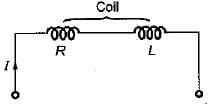A coil acts as open circuit i.e I = 0 if R = ∞  or L = 0 (so that XL = 0Ω).

Test: Capacitors & Inductors- 2 - Question 18

Consider the following statements regarding an inductor:
1. An inductor is a sort of short circuit to dc.
2. An inductor is a sort of open circuit to dc.
3. An inductor never dissipates energy but only stores it.
4. A finite amount of energy can be stored in an inductor even if voltage across it is zero, such as when the current through it is constant.
Q. Which of the above statements is/are not correct?

Detailed Solution for Test: Capacitors & Inductors- 2 - Question 18

An inductor acts as open circuit to dc (as f = 0 for dc so that XL = 0Ω).

Test: Capacitors & Inductors- 2 - Question 19

Assertion (A): A capacitor can store charge.
Reason (R): The plates of the condenser are conductive.

Test: Capacitors & Inductors- 2 - Question 20

Assertion (A): It is impossible to change the current through an inductor by finite amount in zero time.
Reason (R): The current through an inductor can be changed by finite amount in zero time only when an infinite voltage is applied across it.

## GATE Electrical Engineering (EE) 2024 Mock Test Series

23 docs|285 tests
Information about Test: Capacitors & Inductors- 2 Page
In this test you can find the Exam questions for Test: Capacitors & Inductors- 2 solved & explained in the simplest way possible. Besides giving Questions and answers for Test: Capacitors & Inductors- 2, EduRev gives you an ample number of Online tests for practice

## GATE Electrical Engineering (EE) 2024 Mock Test Series

23 docs|285 tests